# Diamond side

The diagonals of the diamond are 18 cm and 14 cm long. Calculate the length of the diamond side.

a =  11.4018 cm

### Step-by-step explanation: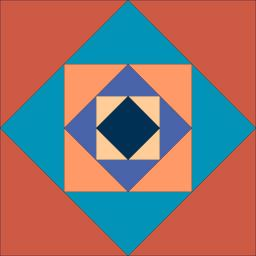We will be pleased if You send us any improvements to this math problem. Thank you!Tips to related online calculators
Pythagorean theorem is the base for the right triangle calculator.
See also our trigonometric triangle calculator.

#### You need to know the following knowledge to solve this word math problem:

We encourage you to watch this tutorial video on this math problem:

## Related math problems and questions:

• Rhombus diagonalsIn the rhombus ABCD are given the sizes of diagonals e = 24 cm; f = 10 cm. Calculate the side length of the diamond and the size of the angles, calculate the content of the diamond
• Diamond diagonalsCalculate the diamonds' diagonals lengths if the diamond area is 156 cm square, and the side length is 13 cm.
• Diamond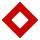Side length of diamond is 35 cm and the length of the diagonal is 56 cm. Calculate the height and length of the second diagonal.
• Diamond and diagonalsA diamond has diagonals f = 8 cm and g = 6 cm long. How long is this diamond perimeter? (Calculate it!)
• Two diagonalsThe diagonals of the diamond EFGH have lengths in the ratio 1: 2. What is the circumference of a rhombus if the longer of the diagonals is 8 cm long?
• Diagonals in diamons/rhombusRhombus ABCD has side length AB = 4 cm and a length of one diagonal of 6.4 cm. Calculate the length of the other diagonal.
• Rhombus and diagonalsThe lengths of the diamond diagonals are e = 48cm, f = 20cm. Calculate the length of its sides.
• Diamond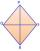Calculate the length of the two diagonals of the diamond if: a = 13 cm v = 12 cm
• Diamond diagonalsFind the diamond diagonal's lengths if the area is 156 cm2 and side is 13 cm long.
• DiagonalsA diagonal of a rhombus is 20 cm long. If it's one side is 26 cm, find the length of the other diagonal.
• Rhombus IVCalculate the length of the diagonals of the rhombus, whose lengths are in the ratio 1: 2 and a rhombus side is 35 cm.
• KiteJohn a kite, which is diamond-shaped. Its diagonals are 60 cm long and 90 cm long. Calculate: a) the diamond side b) how much paper John needs to make a kite if he needs a paper on both sides and needs 5% of the paper for bending.
• The diagonals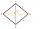The diagonals of the KOSA diamond are 20 cm and 48 cm long. Calculate the circumference of the diamond in centimeters rounded to one unit.
• RhombusIt is given a rhombus of side length a = 19 cm. Touchpoints of inscribed circle divided his sides into sections a1 = 5 cm and a2 = 14 cm. Calculate the radius r of the circle and the length of the diagonals of the rhombus.
• DiagonalsGiven a rhombus ABCD with a diagonalsl length of 8 cm and 12 cm. Calculate the side length and content of the rhombus.
• Diamond diagonalsCalculate the diamond's diagonal lengths if its content is 156 cm2 and the side length is 13 cm.
• Diamond ABCD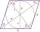In the diamond ABCD, the diagonal e = 24 cm and the size of angle SAB is 28 degrees, where S is the intersection of the diagonals. Calculate the circumference of the diamond.